# CSVTU Exam Question Papers-Engineering Mechanics–Nov-Dec-2009

BE (1st year)

Examination, Nov-Dec-2009

Engineering Mechanics

Unit-I

Q. 1. Part-a is compulsory. Attempt any two parts.from b, c and d.

(a) The force F – of magnitude 500 N is to be resolved into two components along lines a-a and b — b. Determine the angle a, knowing that the component of F along line a — a is to be 400 N as shown in Fig.:-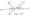(b) Two loads are applied as shown in Fig 1(b),to the end C o1 boom BC. Determine the tension in cable AC, knowing the the resultant of three forces, exerted at C must be directec along BC.

Fig;-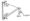(c) A prismatic bar AB of weight W is resting Against a smooth verticp wall at A and is supported Oh a small roller at the point D. If a vertical force F is applied at the end B, find the position of equilibrium as defin by the angle G. Assume AB = e.

Fig:-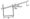(d) A leaver AB is hinged at C and attached to a control cable A. If the leaver is subjected at B to a 400 N horizontal force. Determine the tension in cable and reaction at C. Take CD= 200mm and BC=150mm.

Fig:-Unit-II

Part-a and b are compulsory, attempt any one from c. and d.

(a) State the condition for which, bending moment diagram sharply increases or decreases at a point of a beam.

(b) What is the perfect truss ? Give mathematical conditions and basic assumptions for perfect truss.

(c) Find the relationship between uniformely distributed load, shear forde and bending moment of a beam.

(d) Find the axial forces in the member BG of the truss supported and loaded as shown in

Fig:-Unit – II

Part — a is compulsory. Attempt any two parts from b, c, and d.

(a) Define the following terminology

(i) Limiting friction.

(ii) Angle of Repose.

(b) A 200 N block rests as shown on a wedge of negligible weight.- Knowing that the coeff. of static friction is 0.30 at all surfaces of contact. Determine the angles for which sliding is. Impending and compute the corresponding value of the normal force exerted on the block by the vertical wall.

Fig:-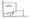(c) A belt 100 mm, wide arid 8 mm thick is transmittimg power a belt speed of 1600 m/minute. The angle of lap fc pulley is 165° and the coeff. of friction is 0.3. The permissible stress in the belt is 2 MN/m2 and the i lass-of the belt is 0.9 kg/m. Find the maximum power that can be transmitte corresponding belt speed.

(d) A homogenous cylinder of weight W rest on a he in contact with a wall. If the coefficient of friction ft surfaces be determine the couple M acting or which-will start counter clockwise’rotation.

Fig:-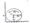Q. 4. (a) State the parallel axis theorem.

(b) Determine the moments of inertia of the shac respect to x-axis by direct integration.

Fig;-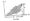(c) Determine the moment of inertia of the area of T-section shown in figure with respect to AB axis.

Fig:-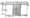(d) For a composite area as shown. Find the relation betweei and r so that x and y will be principal axes.

Fig:-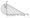Q.5. (a) State the D’Alembert’s Principle.

(d) In a police investigation of tyre marks, it was concluded that a car while in a motion along a straight level road skidded for a total of 60 meters, after the brakes were applied. If the coefficient of fiction between the typer and the pavement is estimated as 0.5 what was the probable speed of the car just before the brake applied.

Or

(C) A’man wishes to move wooden box of 1 meter cube to a distance of 5 m with the least amount of work. If the block weighs 1 KN and the coefficient of friction is 0.3, find whether he should tip it or slide it.